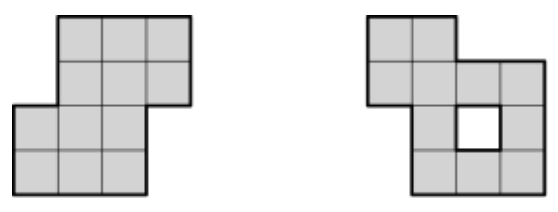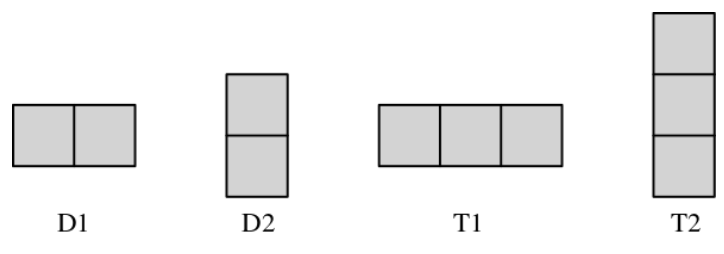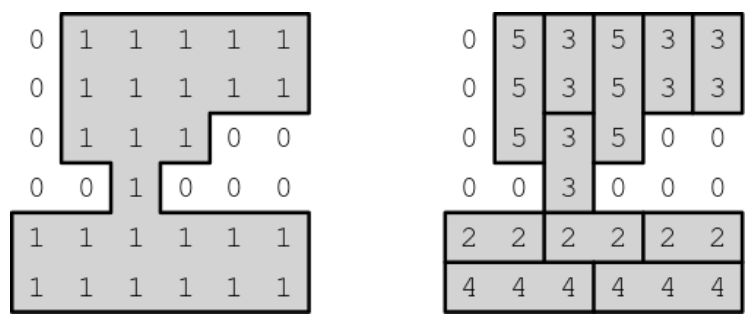시간 제한메모리 제한제출정답맞힌 사람정답 비율
1.5 초 512 MB49241448.276%

## 문제

A polyomino is a plane geometric figure formed by joining one or more unit squares edge to edge. The figure below shows two examples of polyominos. Each of the squares contained in a polyomino is called a cell, and two cells sharing an edge are called neighbors of each other. Note that the number of neighbors of a cell can be 0, 1, 2, 3 and 4. A polyomino P is called connected if every pair of cells (a, b) in P has a path connecting neighboring cells from a to b, and P is called simply connected if P is connected and does not contain any "hole". In the figure below, the left one is simply connected but the right one is not. We will deal with a simply connected polyomino P such that every cell contained in P has two or more neighbors.A tiling of a polyomino P is a tessellation (covering using geometric shapes with no overlaps and no gaps) of P by translated copies of D1, D2, T1, and T2, where D1 (resp. D2) is a polyomino formed by joining two unit squares horizontally (resp. vertically), and T1 (resp. T2) is a polyomino formed by joining three unit squares horizontally (resp. vertically). The figure below shows D1, D2, T1, and T2. According to the shape of P, a tiling of P may or may not exist.To represent a polyomino P, we assume that P is contained in an n × n unit square grid. We label each unit square s in the grid as 1 if s is a cell of P, or 0 otherwise. Then, the unit square grid containing P can be represented by an n × n matrix of 0's and 1's. A tiling of P can also be represented by an n × n matrix of integers as follows. If a cell of P is covered by a copy of D1 or D2, then we label the cell as 2 or 3, respectively. If a cell of P is covered by T1 or T2, then we label the cell as 4 or 5, respectively. The figure below shows an example of tiling and its representation.Given a simply connected polyomino P such that every cell contained in P has two or more neighbors represented by an n × n matrix of 0's and 1's, write a program that outputs a tiling of P, if it exists.

## 입력

Your program is to read from standard input. The input starts with a line containing an integer 2 ≤ n ≤ 1,000, where n is the number of rows and columns of the unit square grid containing a polyomino P. Each of the following n lines contains n many 0's and 1's, and 1 denotes that the square is a cell of P. The polyomino P is simply connected and every cell contained in P has two or more neighbors.

## 출력

Your program is to write to standard output. If there is a tiling of P, print the tiling of P using n lines. Each of the n lines contains n integers from 0 to 5. A 0 represents that the square is not a cell of P. A 2 represents that the square is a cell of P, and is covered by D1. Similarly, a 3, 4, or 5 represents that the square is a cell of P, and is covered by D2, T1, or T2, respectively. If there is no possible tiling of P, then print -1.

## 예제 입력 1

3
011
111
111


## 예제 출력 1

022
322
322


## 예제 입력 2

6
011111
011111
011100
001000
111111
111111


## 예제 출력 2

053533
053533
053500
003000
222222
444444